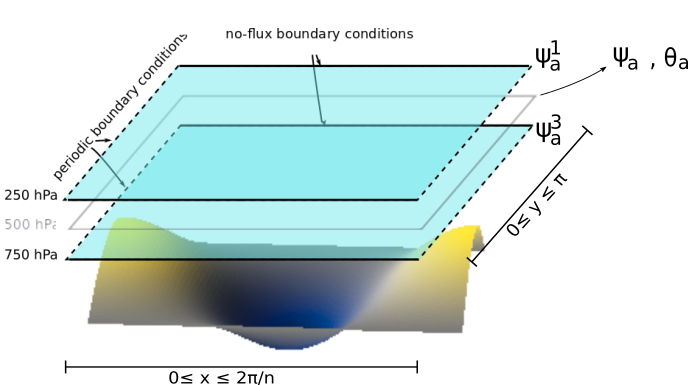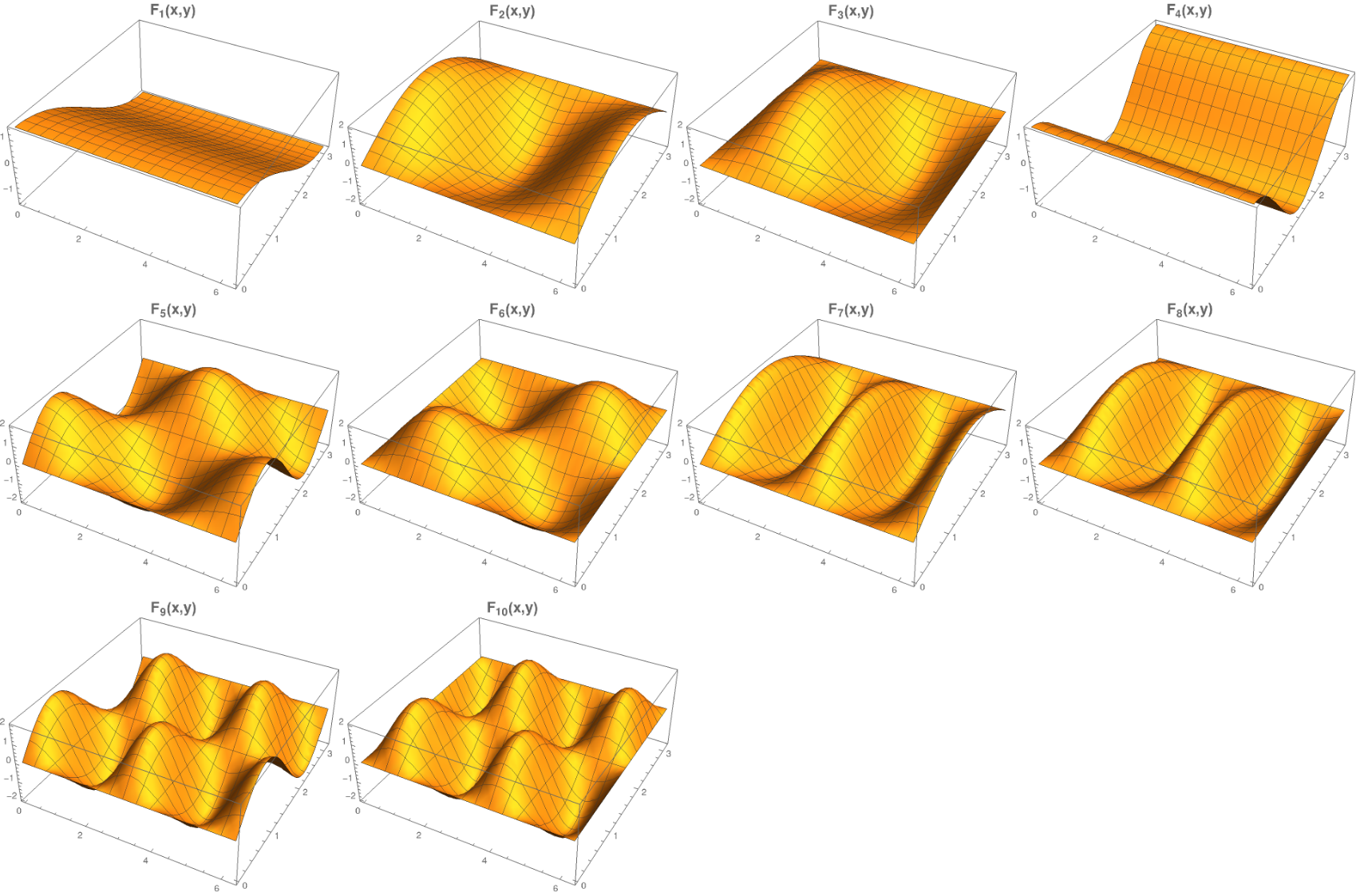# Model with an orography and a temperature profile

An orography and a mean potential temperature profile can be added to the system. The resulting model is described in detail in [CS80] and [RP82].

## Friction with the ground

The atmosphere is coupled to the orography through mechanical coupling (friction).Sketch of the atmospheric model layers with a simple orography. The domain ($$\beta$$-plane) nondimensional zonal and meridional coordinates are labeled as the $$x$$ and $$y$$ variables.

It transforms the equations in Atmospheric component into

$\begin{split}\frac{\partial}{\partial t} \left(\nabla^2 \psi^1_{\rm a}\right)+ J(\psi^1_{\rm a}, \nabla^2 \psi^1_{\rm a})+ \beta \frac{\partial \psi^1_{\rm a}}{\partial x} & = -k'_d \nabla^2 (\psi^1_{\rm a}-\psi^3_{\rm a})+ \frac{f_0}{\Delta p} \omega \nonumber \\ \frac{\partial}{\partial t} \left( \nabla^2 \psi^3_{\rm a} \right) + J(\psi^3_{\rm a}, \nabla^2 \psi^3_{\rm a}) + J(\psi^3_{\rm a}, f_0 \, h/H_{\rm a}) + \beta \frac{\partial \psi^3_{\rm a}}{\partial x} & = +k'_d \nabla^2 (\psi^1_{\rm a}-\psi^3_{\rm a}) - k_d \nabla^2 \psi^3_{\rm a} - \frac{f_0}{\Delta p} \omega \nonumber \\\end{split}$

where $$H_{\rm a}$$ is the characteristic depth of each fluid layer and the parameter $$k_d$$ (kd) quantifies the friction between the atmosphere and the surface. $$h$$ is the topographic height field.

## Mid-layer equations and the thermal wind relation

The equations governing the time evolution of the mean and shear streamfunctions $$\psi_{\rm a} = (\psi^1_{\rm a} + \psi^3_{\rm a})/2$$ and $$\theta_{\rm a} = (\psi^1_{\rm a} - \psi^3_{\rm a})/2$$ is given by

$\begin{split}&\frac{\partial}{\partial t} \left(\nabla^2 \psi_{\rm a}\right) + J(\psi_{\rm a}, \nabla^2 \psi_{\rm a}) + J(\theta_{\rm a}, \nabla^2 \theta_{\rm a}) + \frac{1}{2} J(\psi_{\rm a} - \theta_{\rm a}, f_0 \, h/H_{\rm a}) + \beta \frac{\partial \psi_{\rm a}}{\partial x} = - \frac{k_d}{2} \nabla^2 (\psi_{\rm a} - \theta_{\rm a}) \\ &\frac{\partial}{\partial t} \left( \nabla^2 \theta_{\rm a} \right) + J(\psi_{\rm a}, \nabla^2 \theta_{\rm a}) + J(\theta_{\rm a}, \nabla^2 \psi_{\rm a}) - \frac{1}{2} J(\psi_{\rm a} - \theta_{\rm a}, f_0 \, h/H_{\rm a}) + \beta \frac{\partial \theta_{\rm a}}{\partial x} \nonumber \\ & \qquad \qquad \qquad \qquad \qquad \qquad = - 2 \, k'_d \nabla^2 \theta_{\rm a} + \frac{k_d}{2} \nabla^2 (\psi_{\rm a} - \theta_{\rm a}) + \frac{f_0}{\Delta p} \omega.\end{split}$

$$\psi_{\rm a}$$ and $$\theta_{\rm a}$$ are often referred to as the barotropic and baroclinic streamfunctions, respectively. On the other hand, the thermodynamic equation governing the mean potential temperature $$T_{\rm a} = (T^1_{\rm a} + T^3_{\rm a})/2$$, where $$T^1_{\rm a}$$ and $$T^3_{\rm a}$$ are the mean potential temperature in respectively the upper and lower layer, is given by [Val06]

$\frac{\partial}{\partial t} T_{\rm a} + J(\psi_{\rm a}, T_{\rm a}) = \frac{\sigma \, \Delta p}{R} \,\omega + h_d \, (T^\ast - T_{\rm a})$

where $$h_d$$ (hd) is the Newtonian cooling coefficient indicating the tendency to return to the equilibrium temperature profile $$T^\ast$$. $$\sigma$$ (sigma) is the static stability of the atmosphere, taken to be constant.

The hydrostatic relation in pressure coordinates is $$(\partial \Phi/\partial p) = -1/\rho_\text{a}$$ with the geopotential height $$\Phi = f_0\;\psi_\text{a}$$ and $$\rho_\text{a}$$ the dry air density. The ideal gas relation $$p=\rho_\text{a} R T_\text{a}$$ and the vertical discretization of the hydrostatic relation at 500 hPa allows to write the spatially dependent atmospheric temperature anomaly $$\delta T_\text{a} = 2f_0\;\theta_\text{a} /R$$ where $$R$$ (rr) is the ideal gas constant. Therefore, upon nondimensionalization, both fields are identified with each other: $$2 \theta_{\rm a} \equiv T_{\rm a}$$ and $$2 \theta^\star \equiv T^\star$$, and the equations above fully describe the system.

The mean streamfunction $$\psi_{\rm a}$$ and temperature $$\theta_{\rm a}$$ can be considered to be the value of these fields at the 500 hPa level.

## Projecting the equations on a set of basis functions

The present model solves the equations above by projecting them onto a set of basis functions, to obtain a system of ordinary differential equations (ODE). This procedure is sometimes called a Galerkin expansion. This basis being finite, the resolution of the model is automatically truncated at the characteristic length of the highest-resolution function of the basis.

Both fields are defined in a zonally periodic channel with no-flux boundary conditions in the meridional direction ($$\partial \cdot /\partial x \equiv 0$$ at the meridional boundaries).

The fields are projected on Fourier modes respecting these boundary conditions:

$\begin{split}&F^A_{P} (x, y) = \sqrt{2}\, \cos(P y), \\ &F^K_{M,P} (x, y) = 2\cos(M nx)\, \sin(P y), \\ &F^L_{H,P} (x, y) = 2\sin(H nx)\, \sin(P y)\end{split}$

with integer values of $$M$$, $$H$$, $$P$$. $$x$$ and $$y$$ are the horizontal adimensionalized coordinates, rescaled by dividing the dimensional coordinates by the characteristic length $$L$$ (L). The model’s domain is then defined by $$(0 \leq x \leq \frac{2\pi}{n}, 0 \leq y \leq \pi)$$, with $$n$$ (n) the aspect ratio between its meridional and zonal extents $$L_y$$ (L_y) and $$L_x$$ (L_x).

To easily manipulate these functions and the coefficients of the fields expansion, we number the basis functions along increasing values of $$M= H$$ and then $$P$$. It allows to write the set as $$\left\{ F_i(x,y); 1 \leq i \leq n_\text{a}\right\}$$ where $$n_{\mathrm{a}}$$ (nmod ) is the number of modes of the spectral expansion.

For example, with $$M=H=1$$ and $$P \in \{1,2\}$$, one obtains the spectral truncation used by [CS80]. The model derived in [RP82] extended this set by two blocks of two functions each, and the resulting set can be specified as $$M,H \in \{1,2\}$$; $$P \in \{1,2\}$$. The corresponding set of basis functions is

$\begin{split}F_1(x,y) & = & \sqrt{2}\, \cos(y), \nonumber \\ F_2(x,y) & = & 2\, \cos(n x)\, \sin(y), \nonumber \\ F_3(x,y) & = & 2\, \sin(n x)\, \sin(y), \nonumber \\ F_4(x,y) & = & \sqrt{2}\, \cos(2y), \nonumber \\ F_5(x,y) & = & 2 \cos(n x) \sin(2y), \nonumber \\ F_6(x,y) & = & 2 \sin(n x) \sin(2y), \nonumber \\ & \vdots & \nonumber\end{split}$

such that

$\nabla^2 F_i(x,y) = -a^2_i F_i(x,y)$

with eigenvalues $$a_i^2 = P_i^2 + n^2 \, M_i^2$$ or $$a_i^2 = P_i^2 + n^2 \, H_i^2$$. These Fourier modes are orthonormal with respect to the inner product

$\frac{n}{2\pi^2}\int_0^\pi\int_0^{2\pi/n} F_i(x,y)\, F_j(x,y)\, \mathrm{d} x \, \mathrm{d} y = \delta_{ij}$

where $$\delta_{ij}$$ is the Kronecker delta.The first 10 basis functions $$F_i$$ evaluated on the nondimensional domain of the model.

The model’s fields can be decomposed as follows:

$\begin{split}\psi_{\rm a}(x,y) & = & \sum_{i=1}^{n_{\mathrm{a}}} \, \psi_{{\rm a},i} \, F_i(x,y) \\ \theta_{\rm a}(x,y) & = & \sum_{i=1}^{n_{\mathrm{a}}} \, \theta_{{\rm a},i} \, F_i(x,y) .\end{split}$

The radiative equilibrium temperature field $$\theta^\star(x,y)$$, the topographic height field $$h(x,y)$$ and the vertical velocity $$\omega(x,y)$$ also have to be decomposed into the eigenfunctions of the Laplacian:

$\begin{split}\theta^\star(x,y) & = & \sum_{i=1}^{n_{\mathrm{a}}} \, \theta^\star_i \, F_i(x,y) \\ h(x,y) & = & \sum_{i=1}^{n_{\mathrm{a}}} \, h_i \, F_i(x,y) \\ \omega(x,y) & = & \sum_{i=1}^{n_{\mathrm{a}}} \, \omega_i \, F_i(x,y) .\end{split}$

These fields can be specified in the model by setting the (non-dimensional) vectors hk and thetas. Note that $$h$$ is scaled by the characteristic height $$H_{\rm a}$$ of each layer, and $$\theta^\star$$ is scaled by $$A f_0^2 L^2$$ (see section below).

## Ordinary differential equations

The fields, parameters and variables are non-dimensionalized by dividing time by $$f_0^{-1}$$ (f0), distance by the characteristic length scale $$L$$ (L), pressure by the difference $$\Delta p$$ (deltap), temperature by $$A f_0^2 L^2$$, and streamfunction by $$L^2 f_0$$. As stated above, a result of this non-dimensionalization is that the field $$\theta_{\rm a}$$ is identified with $$T_{\rm a}$$: $$\theta_{\rm a} \equiv T_{\rm a}$$.

The ordinary differential equations of the truncated model are:

$\begin{split}\dot\psi_{{\rm a},i} & = & - a_{i,i}^{-1} \sum_{j,m = 1}^{n_{\mathrm{a}}} b_{i, j, m} \left(\psi_{{\rm a},j}\, \psi_{{\rm a},m} + \theta_{{\rm a},j}\, \theta_{{\rm a},m}\right) - \frac{a_{i,i}^{-1}}{2} \sum_{j,m = 1}^{n_{\mathrm{a}}} g_{i, j, m} \, h_m \left(\psi_{{\rm a},j}-\theta_{{\rm a},j}\right) \nonumber \\ & & \qquad \qquad \qquad \qquad - \beta\, a_{i,i}^{-1} \, \sum_{j=1}^{n_{\mathrm{a}}} \, c_{i, j} \, \psi_{{\rm a},j} - \frac{k_d}{2} \left(\psi_{{\rm a},i} - \theta_{{\rm a},i}\right) \\ \dot\theta_{\rm{a},i} & = & - a_{i,i}^{-1} \sum_{j,m = 1}^{n_{\mathrm{a}}} b_{i, j, m} \left(\psi_{{\rm a},j}\, \theta_{{\rm a},m} + \theta_{{\rm a},j}\, \psi_{{\rm a},m}\right) + \frac{a_{i,i}^{-1}}{2} \sum_{j,m = 1}^{n_{\mathrm{a}}} g_{i, j, m} \, h_m \left(\psi_{{\rm a},j}-\theta_{{\rm a},j}\right) \nonumber \\ & & \qquad \qquad \qquad \qquad - \beta\, a_{i,i}^{-1} \, \sum_{j=1}^{n_{\mathrm{a}}} \, c_{i, j} \, \theta_{{\rm a},j} + \frac{k_d}{2} \left(\psi_{{\rm a},i} - \theta_{{\rm a},i}\right) -2 \, k'_d \, \theta_{{\rm a},i} + a_{i,i}^{-1} \, \omega_i \\ \dot\theta_{\rm{a},i} & = & - \sum_{j,m = 1}^{n_{\mathrm{a}}} g_{i, j, m} \, \psi_{{\rm a},j}\, \theta_{{\rm a},m} + \frac{\sigma}{2} \, \omega_i + h_d \, \left(\theta^\ast_i - \theta_{{\rm a},i}\right)\end{split}$

where the parameters values have been replaced by their non-dimensional ones. The coefficients $$a_{i,j}$$, $$g_{i, j, m}$$, $$b_{i, j, m}$$ and $$c_{i, j}$$ are the inner products of the Fourier modes $$F_i$$:

$\begin{split}a_{i,j} & = & \frac{n}{2\pi^2}\int_0^\pi\int_0^{2\pi/n} F_i(x,y)\, \nabla^2 F_j(x,y)\, \mathrm{d} x \, \mathrm{d} y = - \delta_{ij} \, a_i^2 \\ g_{i, j, m} & = & \frac{n}{2\pi^2}\int_0^\pi\int_0^{2\pi/n} F_i(x,y)\, J\left(F_j(x,y), F_m(x,y)\right) \, \mathrm{d} x \, \mathrm{d} y \\ b_{i, j, m} & = & \frac{n}{2\pi^2}\int_0^\pi\int_0^{2\pi/n} F_i(x,y)\, J\left(F_j(x,y), \nabla^2 F_m(x,y)\right) \, \mathrm{d} x \, \mathrm{d} y \\ c_{i, j} & = & \frac{n}{2\pi^2}\int_0^\pi\int_0^{2\pi/n} F_i(x,y)\, \frac{\partial}{\partial x} F_j(x,y) \, \mathrm{d} x \, \mathrm{d} y\end{split}$

These inner products are computed according to formulas found in [CT87] and stored in an object derived from the AtmosphericInnerProducts class.

The vertical velocity $$\omega_i$$ can be eliminated, leading to the final equations

$\begin{split}\dot\psi_{{\rm a},i} & = & - a_{i,i}^{-1} \sum_{j,m = 1}^{n_{\mathrm{a}}} b_{i, j, m} \left(\psi_{{\rm a},j}\, \psi_{{\rm a},m} + \theta_{{\rm a},j}\, \theta_{{\rm a},m}\right) - \frac{a_{i,i}^{-1}}{2} \sum_{j,m = 1}^{n_{\mathrm{a}}} g_{i, j, m} \, h_m \left(\psi_{{\rm a},j}-\theta_{{\rm a},j}\right) \nonumber \\ & & \qquad \qquad \qquad \qquad - \beta\, a_{i,i}^{-1} \, \sum_{j=1}^{n_{\mathrm{a}}} \, c_{i, j} \, \psi_{{\rm a},j} - \frac{k_d}{2} \left(\psi_{{\rm a},i} - \theta_{{\rm a},i}\right) \\ \dot\theta_{{\rm a},i} & = & \frac{\sigma/2}{a_{i,i} \,\sigma/2 - 1} \left\{ - \sum_{j,m = 1}^{n_{\mathrm{a}}} b_{i, j, m} \left(\psi_{{\rm a},j}\, \theta_{{\rm a},m} + \theta_{{\rm a},j}\, \psi_{{\rm a},m}\right) + \frac{1}{2} \sum_{j,m = 1}^{n_{\mathrm{a}}} g_{i, j, m} \, h_m \left(\psi_{{\rm a},j}-\theta_{{\rm a},j}\right) \right. \nonumber \\ & & \quad - \left. \beta\, \, \sum_{j=1}^{n_{\mathrm{a}}} \, c_{i, j} \, \theta_{{\rm a},j} + \frac{k_d}{2} \, a_{i,i} \left(\psi_{{\rm a},i} - \theta_{{\rm a},i}\right) -2 \, k'_d \, a_{i,i} \, \theta_{{\rm a},i} \right\} \nonumber \\ & & + \frac{1}{a_{i,i} \,\sigma/2 - 1} \left\{ \sum_{j,m = 1}^{n_{\mathrm{a}}} g_{i, j, m} \, \psi_{{\rm a},j}\, \theta_{{\rm a},m} - h_d \, \left(\theta^\ast_i - \theta_{{\rm a},i}\right)\right\}\end{split}$

that are implemented in with a tensorial contraction:

$\frac{\text{d}\eta_{{\rm{a},i}}}{\text{d}t} = \sum_{j, k=0}^{2 n_\mathrm{a}} \mathcal{T}_{i,j,k} \; \eta_{{\rm a},j} \; \eta_{{\rm a},k}$

with $$\boldsymbol{\eta_{\mathrm{a}}} = (\psi_{{\rm a},1}, \ldots, \psi_{{\rm a},n_\mathrm{a}}, \theta_{{\rm a},1}, \ldots, \theta_{{\rm a},n_\mathrm{a}})$$, as described in the Code Description. The tensor $$\mathcal{T}$$ is computed and stored in the QgsTensor.

## Example

An example about how to setup the model to use this model version is shown in Recovering the result of Reinhold and Pierrehumbert (1982).